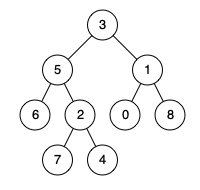# 236. Lowest Common Ancestor of a Binary Tree (Medium)

https://leetcode.com/problems/lowest-common-ancestor-of-a-binary-tree/

Given a binary tree, find the lowest common ancestor (LCA) of two given nodes in the tree.

According to the definition of LCA on Wikipedia: “The lowest common ancestor is defined between two nodes p and q as the lowest node in T that has both p and q as descendants (where we allow a node to be a descendant of itself).”

Given the following binary tree:  root = [3,5,1,6,2,0,8,null,null,7,4]Example 1:

```Input: root = [3,5,1,6,2,0,8,null,null,7,4], p = 5, q = 1
Output: 3
Explanation: The LCA of nodes `5` and `1` is `3.`
```

Example 2:

```Input: root = [3,5,1,6,2,0,8,null,null,7,4], p = 5, q = 4
Output: 5
Explanation: The LCA of nodes `5` and `4` is `5`, since a node can be a descendant of itself according to the LCA definition.
```

Note:

• All of the nodes' values will be unique.
• p and q are different and both values will exist in the binary tree.

## Solutions

### 1.

``````class Solution {
private TreeNode ans;

public Solution() {
// Variable to store LCA node.
this.ans = null;
}

private boolean recurseTree(TreeNode currentNode, TreeNode p, TreeNode q) {
// If reached the end of a branch, return false.
if (currentNode == null) {
return false;
}

// Left Recursion. If left recursion find either node, set left = 1 else 0
int left = this.recurseTree(currentNode.left, p, q) ? 1 : 0;

// Right Recursion
int right = this.recurseTree(currentNode.right, p, q) ? 1 : 0;

// If the current node is one of p or q, or p and q are the same node
int mid = (currentNode.val == p.val) ? 1 : 0;
mid += (currentNode.val == q.val) ? 1 : 0;

// Both nodes are found under node 'currentNode', there are four situations
// 1. left and right
// 2. left and mid
// 3. right and mid
// 4. mid and mid
if (mid + left + right >= 2) {
// Only the lowest parent can set this value, which means only the direct
// parent can make the sum mid + left + right >= 2.
// So here we don't have to check if this.ans is null;
this.ans = currentNode;
}

// Return true if any one of the three bool values is True.
return (mid + left + right > 0);
}

public TreeNode lowestCommonAncestor(TreeNode root, TreeNode p, TreeNode q) {
// Traverse the tree
this.recurseTree(root, p, q);
return this.ans;
}
}
``````

### 2.

``````class Solution {

// This solution does not take one special situation into consideration. That is p
// and q are the same node.

private TreeNode ans;

public Solution() {
// Variable to store LCA node.
this.ans = null;
}

private boolean recurseTree(TreeNode currentNode, TreeNode p, TreeNode q) {

// If reached the end of a branch, return false.
if (currentNode == null) {
return false;
}

// Left Recursion. If left recursion returns true, set left = 1 else 0
int left = this.recurseTree(currentNode.left, p, q) ? 1 : 0;

// Right Recursion
int right = this.recurseTree(currentNode.right, p, q) ? 1 : 0;

// If the current node is one of p or q
// It is better to compare the value instead of the object.
int mid = (currentNode == p || currentNode == q) ? 1 : 0;

// If any two of the flags left, right or mid become True
if (mid + left + right >= 2) {
this.ans = currentNode;
}

// Return true if any one of the three bool values is True.
return (mid + left + right > 0);
}

public TreeNode lowestCommonAncestor(TreeNode root, TreeNode p, TreeNode q) {
// Traverse the tree
this.recurseTree(root, p, q);
return this.ans;
}
}
``````